Select Page
Tags: , , ,

One of the main objectives in robotics is to make things move on their own. To give motion to a robot, electrical motors are used such as stepper motors especially when precision is required with an open-loop control.

prerequisite: Programming with Arduino

## Material

• Computer
• Arduino UNO
• USB cable
• ULN2003APG x1 or H-Bridge SN754410NE x1
• Stepper x1
• jumper cables

## Steppers

Steppers have several phases which if energized with the correct sequence of impulsions can rotate in discrete steps. As the sequence to move one step at a time is known, the position of the rotor can be kept in memory and thus control precisely the motor without sensor.There are two main types of steppers unipolar (5 or 6 wires) and bipolar (4 wires) which have different characteristics such as the number of steps per revolution. Those characteristics should be known and implemented in the code to be able to control the stepper correctly.

## Transistor

To control steppers, both transistor network or H-bridge can be used to apply the correct sequence of impulsions to the coils or phases of the stepper.

For the unipolar example, we used transistor network ULN2003A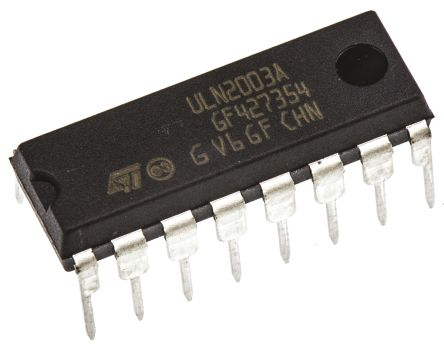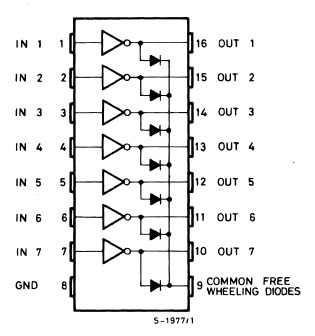For the bipolar example, we used an H-bridge, SN754410NE.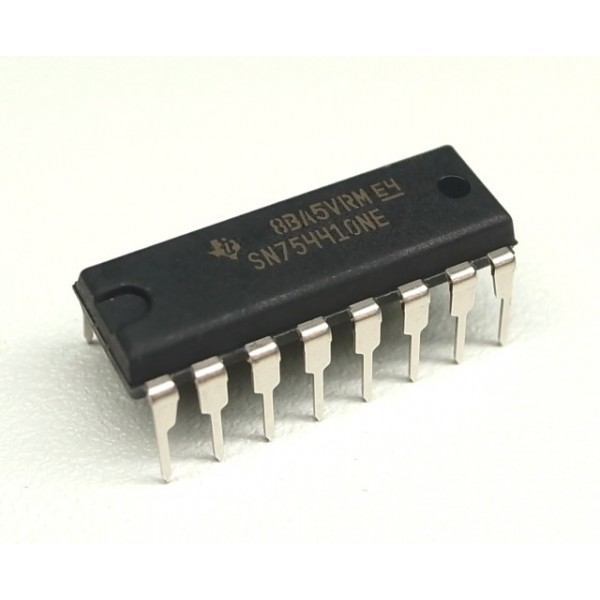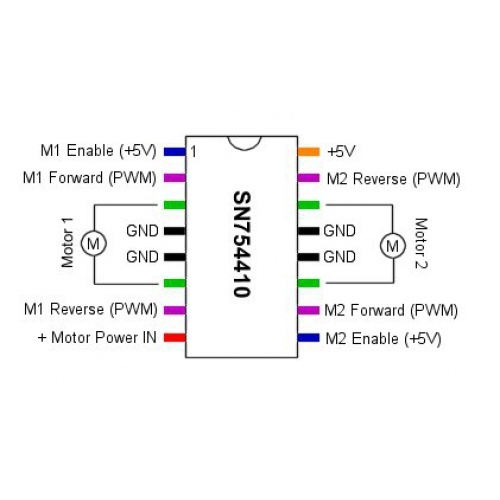## Example with stepper 28BYJ-48

### Schematics

Steppers required a power supply of 3V, 5V or more. External power supply should be preferred especially if the motor requires more than 5V. In this case, the stepper can be powered with the pin 5V of the board and the Arduino can be powered via the USB cable.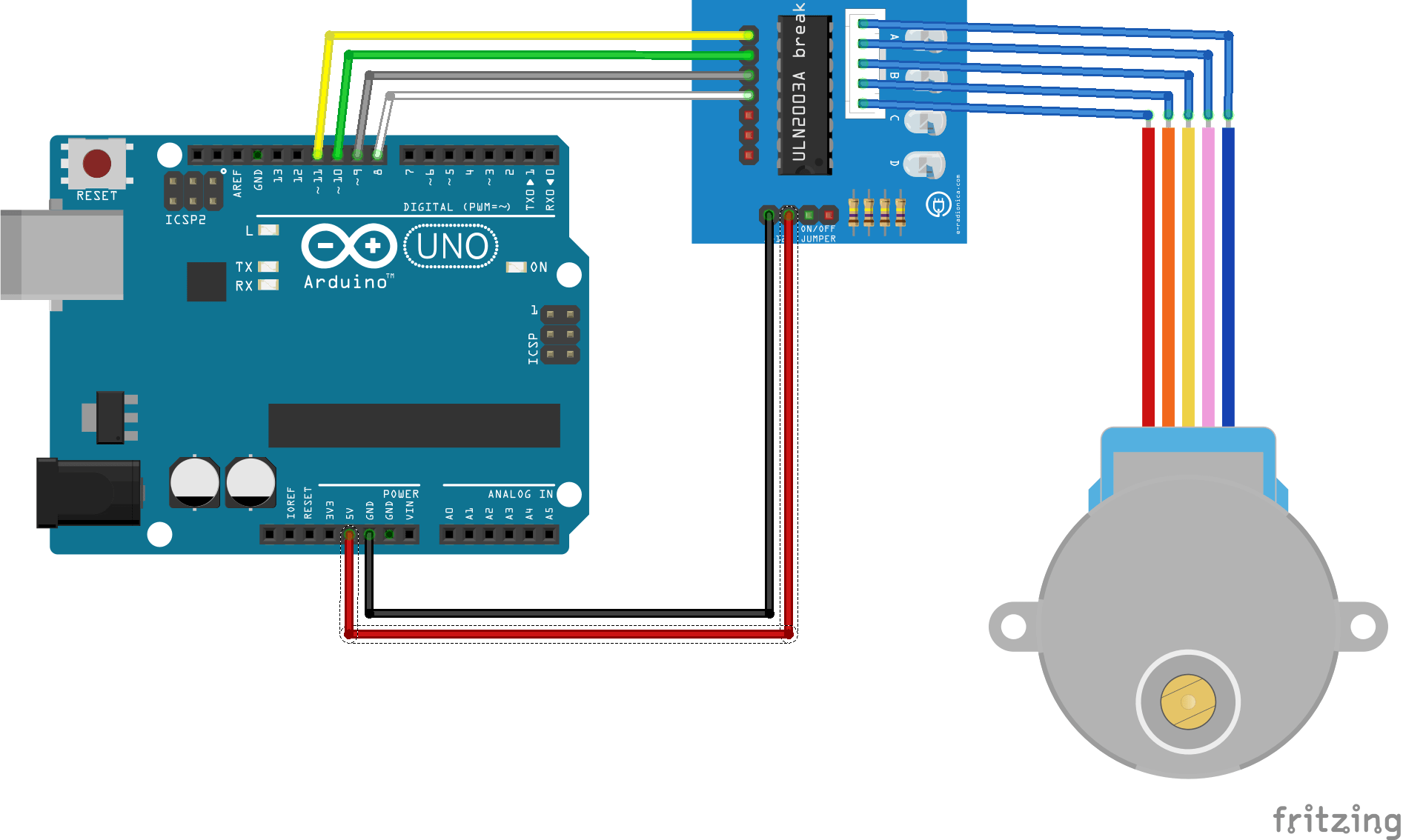### Code

To control a unipolar motor we used a 8-step sequence. The stepper 28BYJ-48 describe a rotation in 2048 steps.

```#define IN1  11
#define IN2  10
#define IN3  9
#define IN4  8
int Steps = 0;
int Direction = 0;
int number_steps=512;//= 2048/4
void setup()
{
Serial.begin(9600);
pinMode(IN1, OUTPUT);
pinMode(IN2, OUTPUT);
pinMode(IN3, OUTPUT);
pinMode(IN4, OUTPUT);
// delay(1000);
}
void loop()
{
//1 rotation counter clockwise
stepper(number_steps);
delay(1000);
//1 rotation clockwise
stepper(-number_steps);
delay(1000);
//Keep track of step number
for(int thisStep=0;thisStep<number_steps;thisStep++){
stepper(1);
}
delay(1000);
for(int thisStep=number_steps;thisStep>0;thisStep--){
stepper(-1);
}
delay(1000);
}
void stepper(double nbStep){
if(nbStep>=0){
Direction=1;
}else{
Direction=0;
nbStep=-nbStep;
}
for (int x=0;x<nbStep*8;x++){
switch(Steps){
case 0:
digitalWrite(IN1, LOW);
digitalWrite(IN2, LOW);
digitalWrite(IN3, LOW);
digitalWrite(IN4, HIGH);
break;
case 1:
digitalWrite(IN1, LOW);
digitalWrite(IN2, LOW);
digitalWrite(IN3, HIGH);
digitalWrite(IN4, HIGH);
break;
case 2:
digitalWrite(IN1, LOW);
digitalWrite(IN2, LOW);
digitalWrite(IN3, HIGH);
digitalWrite(IN4, LOW);
break;
case 3:
digitalWrite(IN1, LOW);
digitalWrite(IN2, HIGH);
digitalWrite(IN3, HIGH);
digitalWrite(IN4, LOW);
break;
case 4:
digitalWrite(IN1, LOW);
digitalWrite(IN2, HIGH);
digitalWrite(IN3, LOW);
digitalWrite(IN4, LOW);
break;
case 5:
digitalWrite(IN1, HIGH);
digitalWrite(IN2, HIGH);
digitalWrite(IN3, LOW);
digitalWrite(IN4, LOW);
break;
case 6:
digitalWrite(IN1, HIGH);
digitalWrite(IN2, LOW);
digitalWrite(IN3, LOW);
digitalWrite(IN4, LOW);
break;
case 7:
digitalWrite(IN1, HIGH);
digitalWrite(IN2, LOW);
digitalWrite(IN3, LOW);
digitalWrite(IN4, HIGH);
break;
default:
digitalWrite(IN1, LOW);
digitalWrite(IN2, LOW);
digitalWrite(IN3, LOW);
digitalWrite(IN4, LOW);
break;
}
delayMicroseconds(1000);
if(Direction==1){ Steps++;}
if(Direction==0){ Steps--; }
if(Steps>7){Steps=0;}
if(Steps<0){Steps=7; }
}
}
```

### Library

A library exists to control motion of stepper motors: Stepper.h.

```#include <Stepper.h>
double stepsPerRevolution = 2048;
Stepper myStepper(stepsPerRevolution, 8, 10, 9, 11);  // Pin inversion to make the library work
void setup() {
myStepper.setSpeed(10);
Serial.begin(9600);
}
void loop() {
// 1 rotation counterclockwise:
Serial.println("counterclockwise");
myStepper.step(stepsPerRevolution);
delay(1000);
// 1 rotation clockwise:
Serial.println("clockwise");
myStepper.step(-stepsPerRevolution);
delay(1000);
}
```

This is the example from the library which had to be modified for this particular stepper (28BYJ-48 130061869) to work. Steppers can be quite different, check the datasheets and play a bit with the parameters to make the code work for your application. (Notes on different types of steppers 28BYJ-48)

## Example with 42HS34

### Schematics

In this case, the stepper can be powered with the pin 3V3 of the board and the Arduino can be powered via the USB cable.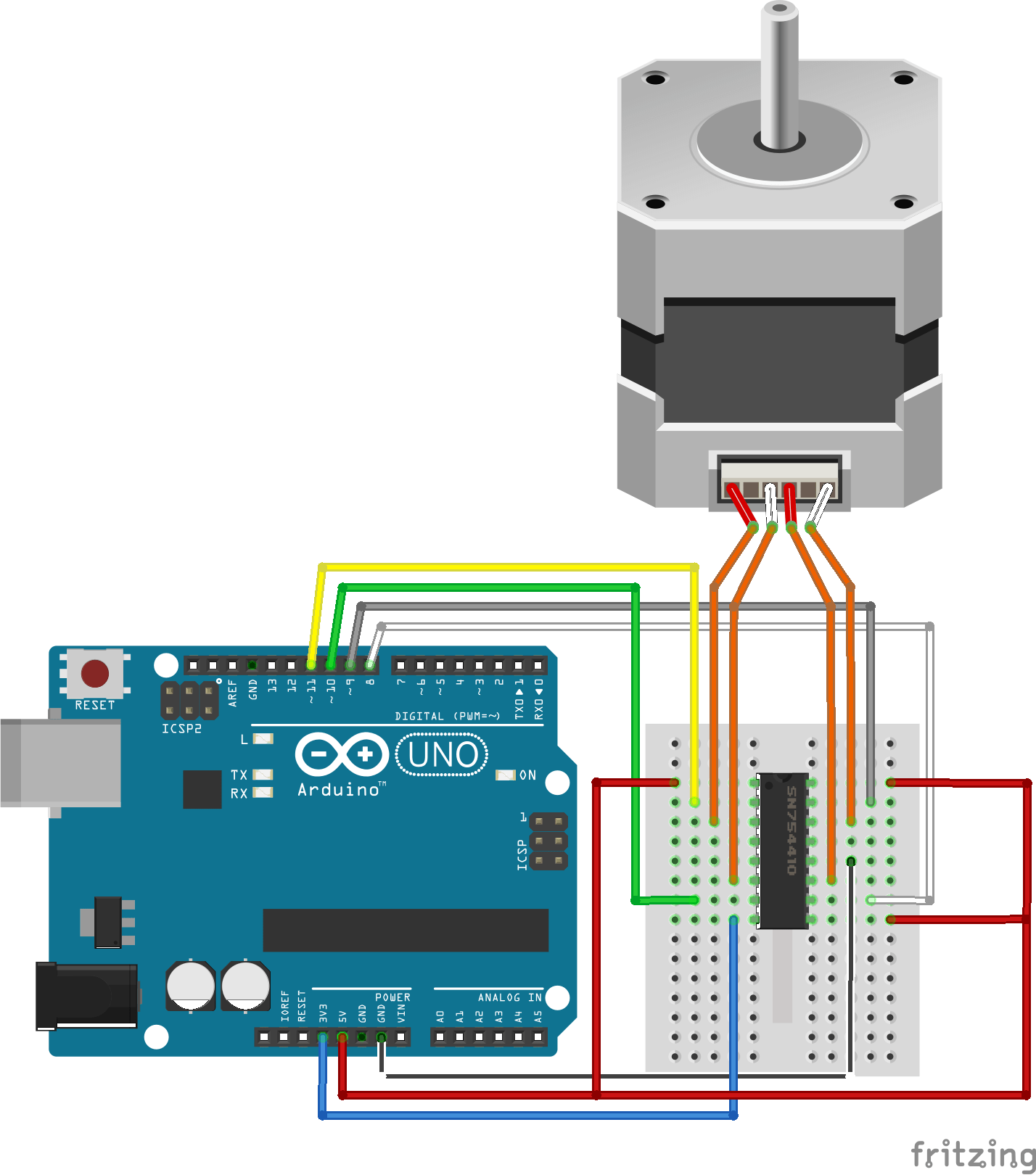### Code

To control a bipolar motor we used a 4-step sequence. The stepper 42HS34 describe a rotation in 200 steps.

```#define IN1  11
#define IN2  10
#define IN3  9
#define IN4  8
int Steps = 0;
int Direction = 0;
int number_steps=50; //= 200/4
void setup() {
Serial.begin(9600);
pinMode(IN1, OUTPUT);
pinMode(IN2, OUTPUT);
pinMode(IN3, OUTPUT);
pinMode(IN4, OUTPUT);
}
void loop()
{
//1 rotation counter clockwise
stepper4(number_steps);
delay(1000);
//1 rotation clockwise
stepper4(-number_steps);
delay(1000);
//Keep track of step number
for(int thisStep=0;thisStep<number_steps;thisStep++){
stepper4(1);
}
delay(1000);
for(int thisStep=number_steps;thisStep>0;thisStep--){
stepper4(-1);
}
delay(1000);
}
void stepper4(double nbStep){
if(nbStep>=0){
Direction=1;
}else{
Direction=0;
nbStep=-nbStep;
}
for (int x=0;x<nbStep*4;x++){
switch (Steps) {
case 0:  // 1010
digitalWrite(IN1, HIGH);
digitalWrite(IN2, LOW);
digitalWrite(IN3, HIGH);
digitalWrite(IN4, LOW);
break;
case 1:  // 0110
digitalWrite(IN1, LOW);
digitalWrite(IN2, HIGH);
digitalWrite(IN3, HIGH);
digitalWrite(IN4, LOW);
break;
case 2:  //0101
digitalWrite(IN1, LOW);
digitalWrite(IN2, HIGH);
digitalWrite(IN3, LOW);
digitalWrite(IN4, HIGH);
break;
case 3:  //1001
digitalWrite(IN1, HIGH);
digitalWrite(IN2, LOW);
digitalWrite(IN3, LOW);
digitalWrite(IN4, HIGH);
break;
}
delayMicroseconds(2000);
if(Direction==1){ Steps++;}
if(Direction==0){ Steps--; }
if(Steps>3){Steps=0;}
if(Steps<0){Steps=3; }
}
}
```

### Library

Code using the library Stepper.h.

```#include <Stepper.h>
const int stepsPerRevolution = 200;
Stepper myStepper(stepsPerRevolution, 8, 9, 10, 11);
void setup() {
myStepper.setSpeed(100);
Serial.begin(9600);
}
void loop() {
// 1 rotation clockwise:
Serial.println("clockwise");
myStepper.step(stepsPerRevolution);
delay(2000);
// 1 rotation counterclockwise:
Serial.println("counterclockwise");
myStepper.step(-stepsPerRevolution);
delay(2000);
}
```